# Gradients of the policy loss in Soft-Actor Critic (SAC)

Source: Deep Learning on Medium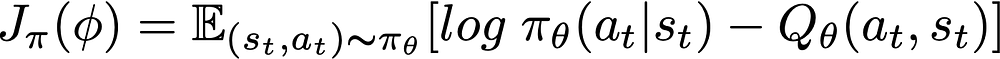Rewriting Eq. 12 from SAC paper before applying the reparameterization trick.

Let’s find the gradient estimator for a more general case and then apply it to the SAC policy loss. The best reference that I’ve found to compute an unbiased gradient estimator with reparameterization trick is . In fact, many of the following equations are from . OK, get ready to dive into some integrals and derivations.

First, let’s define the general objective function that we want to compute an unbiased gradient estimator for: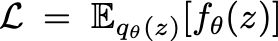Eq. 5 from . Z is a continuous one-dimensional random variable and q is a distribution.

We would like to compute the gradient of L w.r.t. θ, as the following equations:

Let’s first expand the expected value equation with an integral and apply the gradient: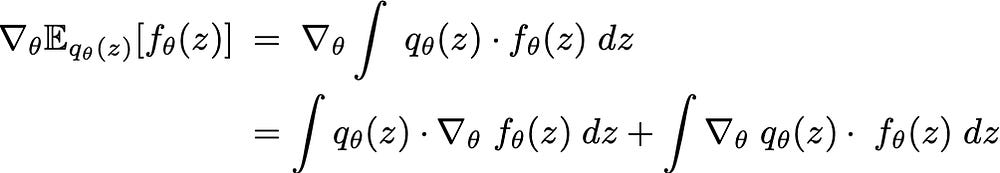Expanding the expected value into its integral and apply the gradient.

The first integral can be easily converted back to an expectation. However, the the gradients of the distribution qθ(z) is intractable. There are two well-known approaches to rectify this, (1) score function method (aka log-derivative trick or REINFORCE) and (2) reparameterization. The Monte Carlo estimates of the latter technique typically yield lower variance than score function method . As such, SAC uses reparameterization to compute the gradients of the distribution qθ(z). The reparameterization trick replace the density function qθ(z) with a fixed distribution that does not depend on θ.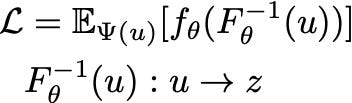Objective function after applying reparameterization. Renamed some of the variable names compared to .

The reparameterization technique pushes all the functions depending on θ inside the expectation. We solved one issue, but another one is born. That is, computing inverse CDF of qθ(z), which for some distributions do not have a simple analytic expression (See  for why we do not use the inverse CDF).

We can fix this problem by using implicit differentiation. We first write the forward CDF formula on which we apply gradients.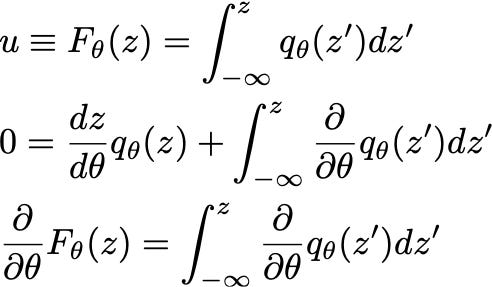Applying gradients on both sides of the first equation and using Leibniz integral rule. Note that, u does not depend on θ, hence its gradient becomes zero.

Using the last three equations, we obtain the golden equation that helps us to compute the gradients of the objective.The golden equation that helps us to derive the gradients of the objective function.

Now we have all the ingredients to compute the gradients of the objective function. Let’s revisit the computation of the second integral and use some calculus techniques as follows: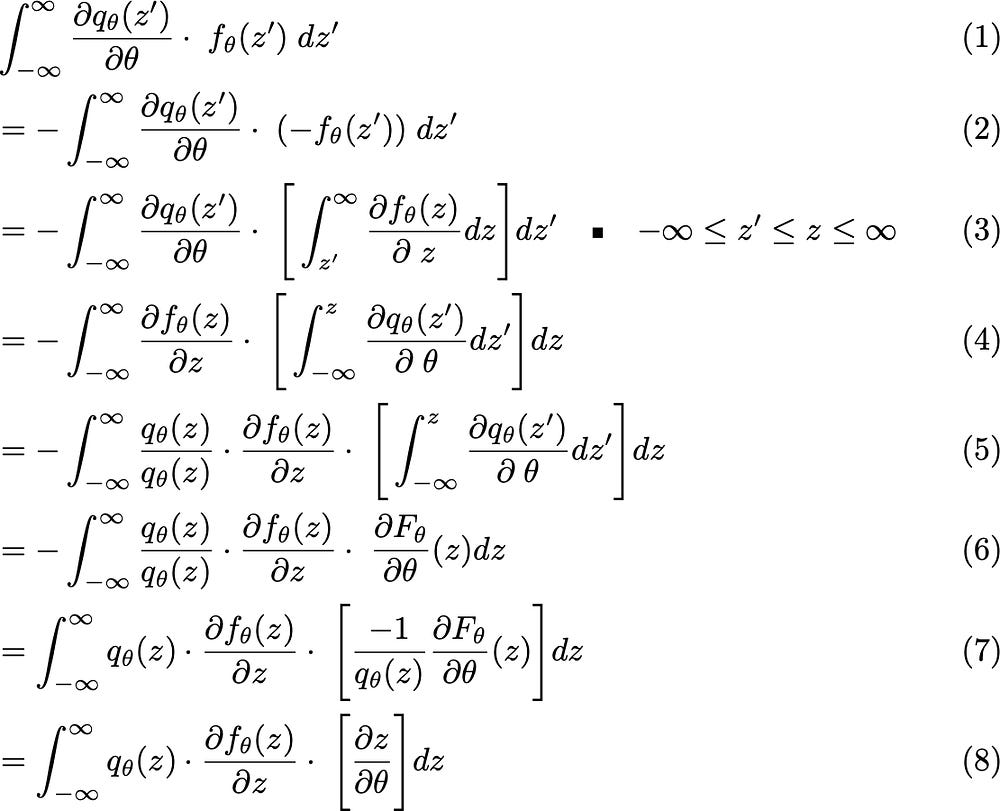The series of equations to calculate the gradients of distribute qθ(z).

Let’s review some of the calculus techniques we used (also you can refer to ). From Eq. (2) to Eq. (3), we just rewrite fθ(z) as the integral of derivative. “We also assume that fθ(z) is sufficiently regular that we can drop the boundary term at infinity”. From Eq. (3) to Eq. (4) we change the order of integration. To better understand how changing the order of integration works in this example, check the inequality I wrote in equation Eq. (3). In Eq. (5) we multiply the equation with 1 (qθ(z)/qθ(z)). I think you should realize by now what we are trying to achieve. We want to transform this integral to one that represents an expected value on qθ(z). Note that, in Eq. (5), we use the definition of gradients on F to obtain Eq. (6). Finally, we use the golden equation to obtain Eq. (8). Is this familiar to you? Yes. You are right. The integral in Eq. (8) represents an expected value on qθ(z).

To wrap up, we can write the gradients of our objective function as follows: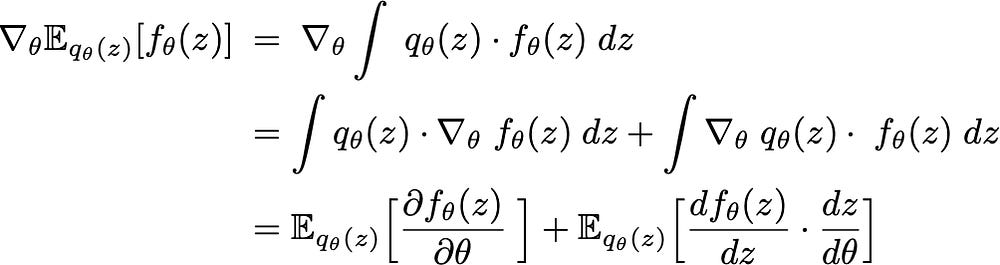The gradients of objective functions in terms of expected values.

Note that, in Eq. 13 , the first and the second term corresponds to the first and the second term of our equation, correspondingly. I hope this article helps others to better understand the paper .